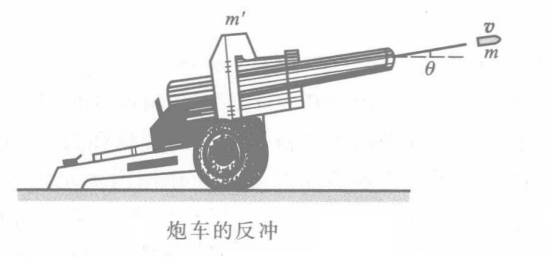$$-m^{\prime} v^{\prime}+m\left(v \cos \theta-v^{\prime}\right)=0$$

$$v^{\prime}=\frac{m}{m+m^{\prime}} v \cos \theta$$

$$m u_x(t)-m^{\prime} v^{\prime}(t)=0$$

$$d=l \cos \theta-D$$

$$D=\left(\frac{m}{m+m^{\prime}}\right) l \cos \theta$$